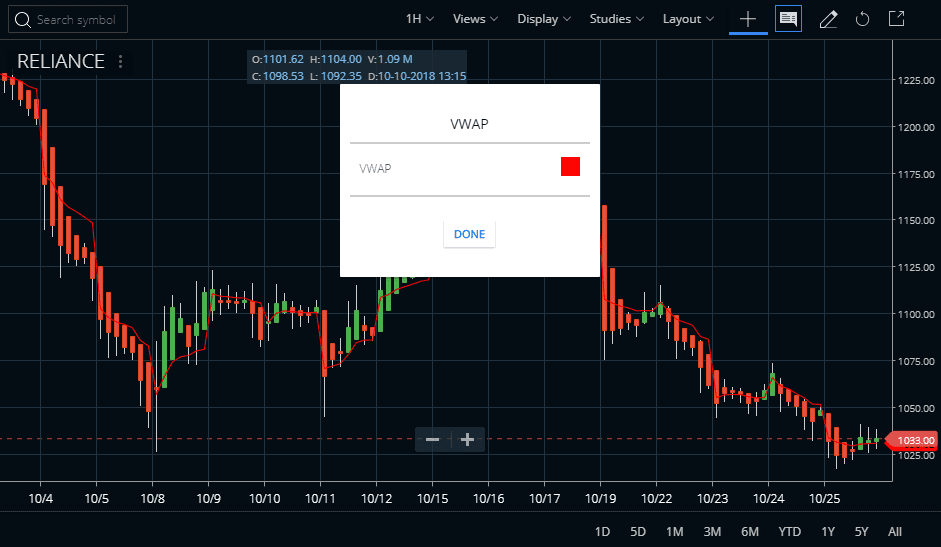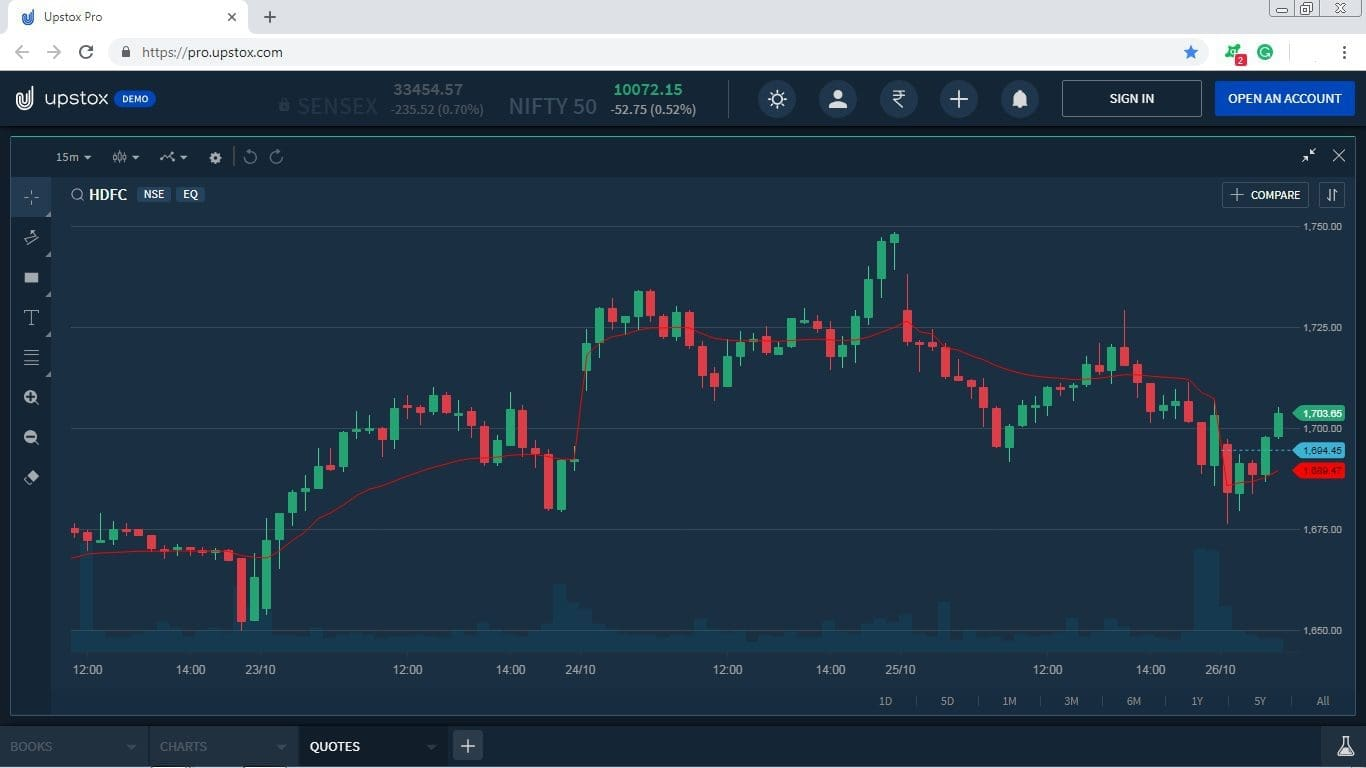# Volume Weighted Average Price Indicator or VWAP

Volume Weighted Average Price indicator is a technical analysis tool. It is used to measure the average price weighted by volume. The volume-weighted average price (VWAP) indicator is mainly used with intraday charts as a way to determine the general direction of intraday prices. VWAP indicator is similar to a moving average when the price is above VWAP, prices are rising and vice versa. This indicator is primarily used by technical analysts to identify market trends.

For further data regarding the volume weighted average price (VWAP) indicator, go to the STUDIES section of Zerodha Kite. This indicator also available in Kite mobile App. In this indicator shown as the VWAP line is red if you want to change this color then you can change it. The traders can also check how we attached the Ultimate Oscillator indicator in Reliance Industries shares price chart. The volume-weighted average price (VWAP) indicator on to any charts has daily, weekly, monthly or intraday time limit.## Description :

VWAP indicator is exactly what it sounds like the average price weighted by volume. Volume Weighted Average Price (VWAP) indicator equals the dollar value of all trading periods divided by the total trading volume for the current day. This indicator is a trading benchmark used especially in pension plans. VWAP indicator is calculated in adding up the dollars traded for every transaction which price multiplied in the number of shares traded and then dividing by the total shares traded for the day. The main theory of this indicator is if the price of a buy trade is lower than the VWAP, it is a good trade. The opposite way is true if the price is higher than the VWAP.

## Calculation :

There are five steps of calculating VWAP indicator :

1. The first step is the Typical Price for the period = [(High + Low + Close)/3)].

2. The second step is to multiply the Typical Price by the period Volume = (Typical Price x Volume).

3. The third step is to create a cumulative total of Typical Price = Cumulative(Typical Price x Volume).

4. The fourth step is to create a cumulative total of Volume = Cumulative(Volume).

5. The last step is to divide the Cumulative Totals.

Volume Weighted Average Price (VWAP) = Cumulative(Typical Price x Volume) / Cumulative (Volume).

## Uses for Volume Weighted Average Price Indicator :

Volume Weighted Average Price (VWAP) indicator is used to identify liquidity points. As a volume-weighted price measure, VWAP indicator reflects price levels weighted by volume. This way can help institutions with large orders. This condition is not to disrupt the market when entering large buy or sell orders. This indicator helps these institutions determine the liquid and illiquid price points for a specific security over a very short time period. VWAP indicator also is used to measure trading efficiency. After buying or selling a security, the traders can compare their price to VWAP values. In this indicator, a buy order executed below the VWAP value will be considered a good fill because the security is to buy at a below average price. As the same way, a sell order executed above the VWAP will be deemed a good fill because it is sold at an above average price.## Chart from Upstox

In order to clarify the particular indicator more specifically, we’ve decided to given examples from the different trading platform. A chart on Volume Weighted Average Price indicator is given below:This is a 15 min chart of HDFC bank. As the chart reflects, when the price crosses the indicator, market trend change. Price downside the indicator is considered as the downtrend and vice versa.

## Example from FYERSWhenever the price breaks the indicator, a new trend arrives in the market.

## Conclusions :

Volume Weighted Average Price (VWAP) indicator serves as a reference point for prices for one day. This indicator is best suited for intraday analysis. The traders can compare current prices with the VWAP values to determine the intraday trend. VWAP indicator also is used to determine relative value. In the Volume Weighted Average Price indicator the VWAP values are relatively low for that day or that specific time for prices below. These values are relatively high for that day or that specific time for prices above. This is a cumulative indicator, which means the number of data points progressively increases throughout the day. In VWAP as an example, on a 1-minute chart, IBM will have 90 data points (minutes) by 11:00 AM, 210 data points by 1:00 PM and 390 data points by the close. The number dramatically increases as the day extends. In Volume Weighted Average Price indicator, this is why VWAP values lag price and this lag increases as the day extends.

Categories: Technical Indicators

+